Study Guide

# Simplifying Rational Expressions

## Simplifying Rational Expressions

A rational expression is just a ratio (fraction) of two polynomials, kinda like a rational number is a ratio of two integers. The word "rational" has "ratio" tucked right in there, so it's easy to remember.

If your first reaction is to run screaming from your computer whenever numbers get too complicated (like, fancier than "1 + 1"), calm down and think "simple." Wrestle down those big, bad rational expressions by putting them in their place—treat 'em like fractions. Just like with fractions, we can write rational expressions down and reduce them to their lowest common denominators.

Here's how you do it. First, factor the numerator and denominator. Then, get rid of any factors that cancel out. Keep everything else.

Yep, that's all there is to it. No screaming necessary.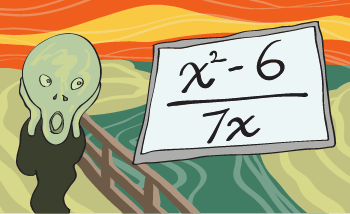If you like things real simple, you can write a rational expression like this: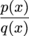...where p(x) and q(x) are different polynomials and q(x) ≠ 0 ('cause we can't divide by 0).

We can play around with all kinds of different rational expressions, but let's start simple. Real simple.

### Sample Problem

Simplify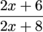.

First, factor both the numerator and denominator. All this means is what multiplier do 2, 6, and 8 share? 2? Yeah.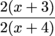This 2 is the factor slated for oblivion.

Now, cancel the common factors.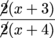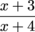Voilà. Looks way simpler than our starting rational expression.

Simplify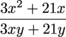.

First, factor the numerator and the denominator.We've got a common factor of 3x in both terms in the numerator, and the denominator has a 3y we can yank out from both terms.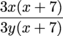Now cancel the common factors.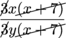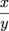Nailed it.

### Sample Problem

Simplify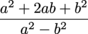.

Factor the numerator and denominator using our fancy polynomial identities.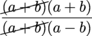Cancel the common factors.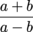Ah, much better.